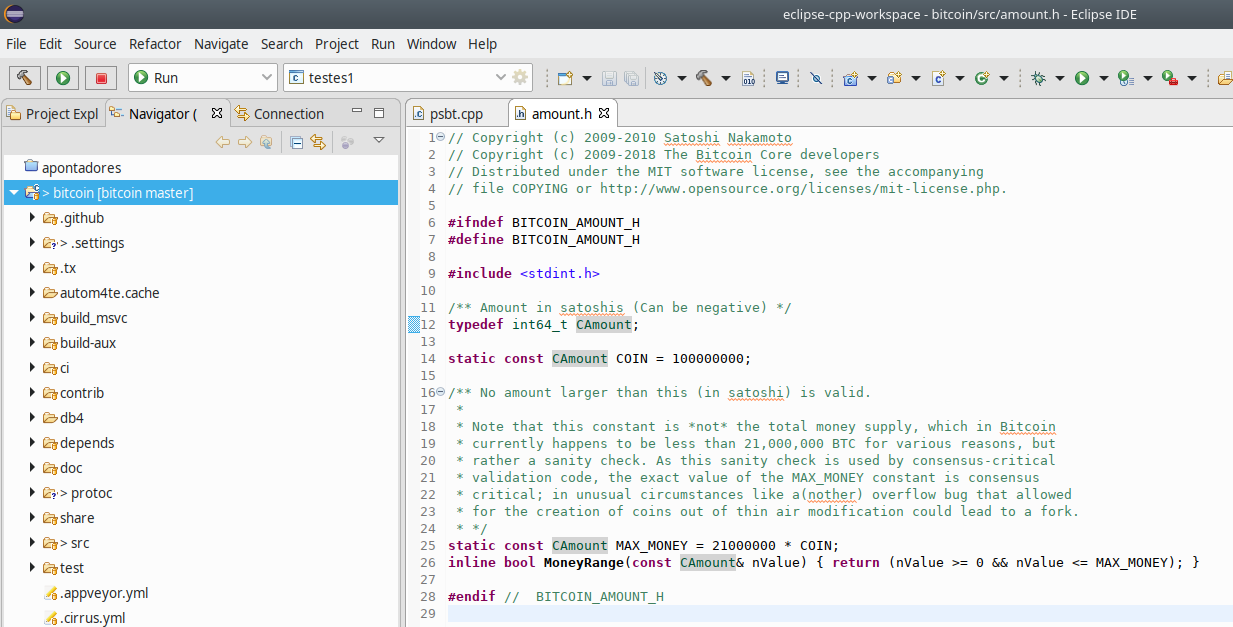# amount.h – Commented Bitcoin Source Codeamount.h is one of simplest source files in Bitcoin Core.

In fact we can paste it in it entirety and discuss it afterwards:

``````/** Amount in satoshis (Can be negative) */
typedef int64_t CAmount;

static const CAmount COIN = 100000000;

/** No amount larger than this (in satoshi) is valid.
*
* Note that this constant is *not* the total money supply, which in Bitcoin
* currently happens to be less than 21,000,000 BTC for various reasons, but
* rather a sanity check. As this sanity check is used by consensus-critical
* validation code, the exact value of the MAX_MONEY constant is consensus
* critical; in unusual circumstances like a(nother) overflow bug that allowed
* for the creation of coins out of thin air modification could lead to a fork.
* */
static const CAmount MAX_MONEY = 21000000 * COIN;
inline bool MoneyRange(const CAmount& nValue) { return (nValue >= 0 && nValue <= MAX_MONEY); }``````

First the `typedef int64_t CAmount` typedef is defined.

To explain this `typedef`, perhaps we should discuss how a Bitcoin is stored in order to understand why a 64 bit integer is enough to represent any Bitcoin amount.

## Bitcoin Numeric Representation

One Bitcoin can be subdivided into 8 decimal places and the largest possible amount of BTC is 21 million (21 with 6 zeroes).

So in total, a Bitcoin amount can take up 16 decimal places: 8 to the left of the dot, 8 to the right.

In Bitcoin Core all amounts are in Satoshis. There is no decimal dot. The system knows that anything under the 8th decimal place is a fraction and everything over that is a whole BTC – there is no dot in the representation, which is why an `int64_t` can store any Bitcoin amount as an integer.

The pattern is somewhat like this: bbbbbbbbssssssss where the b’s are whole BTC and s’s are Satoshis. When the system encounters a CAmount of 7898 for example, that means 7898 Satoshi or 0.00007898 Bitcoin. 100007898 would mean 1 BTC and 7898 Satoshi or 1.00007898 BTC. Except in the source code representation you’ll never find a decimal dot.

As you work with Bitcoin you get used to visualizing the 8 positions before and after the dot.

Magnitude of powers of 2 can be relatively approximated to powers of 10 by dividing the logarithm by 3.

A 64 bit signed integer can take 2^63 – 1 different values.

If we divide 63 by 3, we get 21.

So a 64 bit integer can store up to roughtly 21 decimal places.

Bitcoin requires 16 – so `int64_t` is more than enough.

CAmount is just a typedef’ed int64_t. You’ll see this type all throughout the source code.

## COIN

Next a COIN is defined to be`static const CAmount COIN = 100000000`

As we just discussed this is a digit 1 just one place to the left of 8 zeroes. Remember 8 zeroes are the fractional places (bbbbbbbbssssssss).

Therefore this defines 1 BTC. It’s used throughout the source code to represent one whole Bitcoin.

## MAX_MONEY

This is simply a ceiling amount that can be used to test for valid amounts.

Anything over `MAX_MONEY` is invalid.

You may think this is unnecessary, but an early Bitcoin hack in fact exploited a bug to generate amounts much larger than `MAX_MONEY`.

As you can see `int64_t` is a signed integer. If you enter a negative number, the leftmost bit will be set in a `int64_t`.

If an integer with the highest bit set is interpreted as an unsigned number (as all Bitcoin are unsigned), you get an astronomic number. This exploit was used to generate billions of BTC in 2010.

If this were to happen today somehow, this number would be compared to MAX_MONEY and it’d be immediately rejected.

## Don’t trust, verify

Lastly `MoneyRange(const CAmount& nValue)` simply applies the rules we’ve just discussed.  Valid Bitcoin amounts must be non-negative and below or equal `MAX_MONEY`